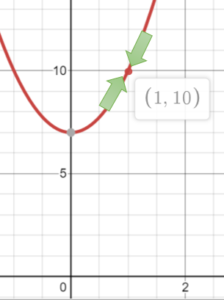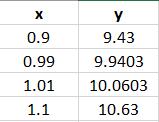# Continuity Test (Calculus)

The continuity test is a set of three conditions that tell you whether a function is continuous at a specific point.

A function is continuous at an x-value of “c” if all of the following conditions are true:

1. The function is defined at c: In other words, if you put the “x” value into the function, you’ll get a real value and not, for example, division by zero or something else that’s undefined.
2. The function approaches the same value from the left and the right. In other words, the limit from above and the limit from below are in agreement.
3. The function approaches the value f(c) from left and right. This is slightly different from #2, which just asks if they approach the same value. This condition states that the “same value” in condition 2 is the value you get when you plug the x-value into the function. In other words, conditions 1 and 2 should equal the same y-value.

## Continuity Test Examples

Example question: Is the function f(x) = 3x2 + 7 continuous at x = 1?

Solution: Check the three conditions given in the definition.

1. Is the function defined at c? Replace “x” in the formula to give:
f(x) = 3(12) + 7 = 10. Yes, the function is defined at x = 1.
2. Does the function approach the same value from the left and the right?. There are a couple of ways to check this:
• Graph the function and check to see if both sides approach the same number.Approaching x = 1 from both sides, both arrows point to the same number (y = 10).

This graph shows that both sides approach f(x) = 16, so the function meets this part of the continuity test.
• You can also create a table of values for small increments close to x = 1:The numbers in the table are getting close to 10 from both sides (from the negative and positive directions).
3. Does the function approach the value f(c) from above and below? In this step, compare the values from Step 1 and Step 2 above. Yes, the two sides meet at y = 10.

This function has passed the continuity test. We can conclude that the function is continuous.

CITE THIS AS:
Stephanie Glen. "Continuity Test (Calculus)" From StatisticsHowTo.com: Elementary Statistics for the rest of us! https://www.statisticshowto.com/continuity-test-calculus/